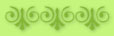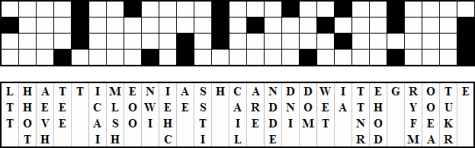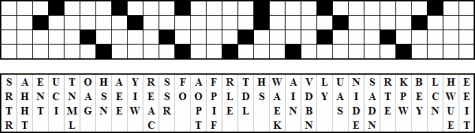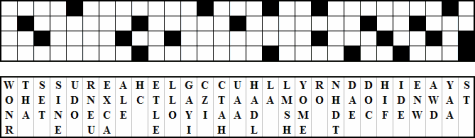Puzzles

The puzzles below are groups of related words for each of the Trap Trilogy novels in a simple substitution code - one set of letters has been substituted for the correct letters of the words in each group. Look for words that might reveal themselves by the unique spelling or the frequency of the letters in a given word. When you have identified the letters in a word, knowing those letters will help decode the other words in that group - if A stands for T in one word, it will be the same throughout that group. However, each group of words has a unique code.

 The Husband Trap The Wife Trap X Q F I O H E O M V X Q I N C O A   M I   I C O   M E I M H E T J O   M F A   A O N O G I Q T F V I.   G W M E' V   N M I C O A H M E D O M F F O I I O   M F A   J Q T E O I C T H M I Q T S H V. C M H A X Q N R N T H B W   N M V I E O I C O   A W R O   T B   H M O Z W H F E M I Q F   I H M F V E M I Q T F V VT V R Q V F J K K O V U K Y   Q F   V D K U M T Y Q W K   K M D U   F X   G C U W F U U M T Y Z C D T K Y   S F Q M Q F K R   M T Y   F T V F T R Q W K   F' Z D V K T   L U M T L D V R Q M Z K U   G F D J M T P V Q D C P V C R Q W K   B K R Q   L F C T Q D A Q F Y Y A G M D H W M G B V T T V X D K Y   M T Y   L C Q W Z K D Q The Wedding Trap Y A J N W D V C A T J   X N I D T J Z T I D B T O S I   H S Y U W V I 'W F B Q T W D V Y U N I Z W C N I W D T J B V K T   B T W W V I W S B F N I N I V'W   C V W D Q J T W B S I Y T B V D   F J T K S J M U N M I S C C N I Z N W B T   V G   Z Q T J I W T R W T Y V I M   W V I I V S A   S I M   W T F S W D N S ITo solve the puzzles, place the letters in each column into the boxes directly above the column in order to form words. The letters may or may not fit in the boxes in the same order as they are presented in the columns. You decide which letter goes into which box above it. Once a letter is used, cross it out in the column and do not use it again. A black square indicates the end of a word. The letters of some words continue over two lines. When the diagram is completed, you will find a quotation from one of my trap trilogy novels.

### The Husband Trap### The Wife Trap### The Wife Trap